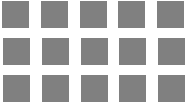Skill 10F Arrays: Arranging Objects in Rows and Columns When learning multiplication concepts and multiplication facts, arrays can be used to help teach the concept and help find the answers prior to successful memorization. Since the array below shows 3 rows of 5 squares, we could say it would be a model for the number sentence 3 x 5 = 15 ( 3 rows of 5 blocks). Typically in math, rows correspond to the first number while columns correspond to the second number. Alicia made an array by arranging square tiles into rows and columns. She used this array to model a number sentence.What number sentence did she model? ______  x  _____  =  _____